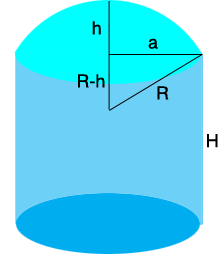SEARCH HOMEMath Central Quandaries & QueriesQuestion from Brandon: I have a tank with a 13' radius that has a domed roof of 3.5' tall How do I figure out the area in SQFT?Hi Brandon,

I drew a diagram of what I think you have.The radius of the tank is $a = 13$ feet and the height of the tank is $h = 3.5$ feet. You didn't tell us the height $H$ of the tank but I expect it is only the area of the dome that you need. I found an expression for the area of the dome or circular cap on the Wolfram MathWorld web site, that's where I got the notation in my diagram. The expression they have is that the area of the dome is

$\pi \left(a^2 + h^2 \right) \mbox{ square feet.}$

For your tank that gives

$\pi \left(13^2 + 3.5^2 \right) = 569.4 \mbox{ square feet.}$

PennyMath Central is supported by the University of Regina and the Imperial Oil Foundation.